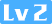Python matplotlab库使用方法及注意事项CuterCorley127 0 0

# 1.Python使用Matplotlib模块时坐标轴标题中文及各种特殊符号显示方法

import matplotlib.pyplot as plt
t = arange(-4*pi, 4*pi, 0.01)
y = sin(t)/t
plt.plot(t, y)
plt.title('www.jb51.net - test')
plt.xlabel(u'\u2103',fontproperties='SimHei')
#在这里，u'\u2103'是摄氏度，前面的u代表unicode，而引号里的内容，是通过在网上查找“℃”这一个符号的unicode编码得到的。这里的“摄氏度”是中文，要显示的话需要在后面加上fontproperties属性即可，这里设置的字体为黑体。
plt.ylabel(u'幅度',fontproperties='SimHei')#也可以直接显示中文。
plt.show()

# 2.将已知折线平滑化或者插值

import numpy as np
from scipy import interpolate
import matplotlib.pyplot as plt
x = np.linspace(-np.pi, np.pi, 10)
y = np.sin(x)
plt.plot(x, y)

f = interpolate.interp1d(x, y, kind='cubic')
nx = np.linspace(-np.pi, np.pi, 30)
ny = f(nx)
plt.plot(nx, ny)

# 3.使用matplotlib的savefig保存图片时不清晰以及不完整的解决办法

savefig(fname, dpi=None, facecolor=‘w’, edgecolor=‘w’,orientation=‘portrait’, papertype=None, format=None,
transparent=False, bbox_inches=None, pad_inches=0.1,frameon=None, metadata=None)

plt.savefig("data/temp.png",dpi=500,bbox_inches = 'tight')#解决图片不清晰，不完整的问题